Factors Affecting Solar Photovoltaic Power Output at Particular Location and Cost Estimation | OMICS International
Journal of Electrical & Electronic Systems

# Factors Affecting Solar Photovoltaic Power Output at Particular Location and Cost Estimation

Nakkela H*

Andhra University College of Engineering, Visakhapatnam, Andhra Pradesh, India

*Corresponding Author:
Nakkela H
Andhra University College of Engineering
Tel:
9177018213
E-mail: [email protected] gmail.com

Received Date: September 29, 2015; Accepted Date: December 22, 2015; Published Date: January 01, 2016

Citation: Nakkela H (2016) Factors Affecting Solar Photovoltaic Power Output at Particular Location and Cost Estimation. J Electr Electron Syst 5:166. doi:10.4172/2332-0796.1000166

Copyright: © 2016 Nakkela H. This is an open-access article distributed under the terms of the Creative Commons Attribution License, which permits unrestricted use, distribution, and reproduction in any medium, provided the original author and source are credited.

Visit for more related articles at Journal of Electrical & Electronic Systems

#### Abstract

Fossil fuels like coal, gas, diesel etc., used for generate electrical energy are in exhaust stage and causes pollution. This necessitates alternative sources of fuels which are renewable and non-pollutant like wind, solar, tidal to generate electrical energy. These renewable fuels cannot be supplied to generate power as required like conventional fuels, as they are naturally available and depends on environmental conditions. Hence they have to be efficiently utilized when they are available. Solar energy is the electromagnetic waves in the form of light which contains packets of energy known as photons. These photons produce electrical energy by the process known photovoltaic effect. In this paper studies are made to utilization of solar energy to produce electrical energy, for this sun-earth angular relation was found using declination angle of earth based on day number, azimuth angle, altitude angle, angle of incidence for particular location i.e., Andhra university, Visakhapatnam with latitude 17.680° N and longitude 83.320° E. Collecting radiation data for the consider site and calculating actual radiation on tilt surface by applying correction to the data collected. Simulation of solar photovoltaic panel using five parameter model of cell considering top Indian manufactured Solar Panels and estimating output DC power by using location Radiation, temperature and wind as input. Comparing output of simulation with on-line simulators like PV watts and off-line software’s SAM, PVs yst. Second part of this paper is estimation of installation cost of solar power plant, cost of generations, Performance ratio, Capacity utilization Factor, LCOE, return of investment, comparing with conventional utility rates.

#### Keywords

Altitude angle; Azimuth angle; Declination angle; Latitude; LCOE; Longitude; PR; PVSYST; PV watts; Radiation data; CUF

#### Introduction

Solar Energy is a free source of energy which is very important to all living beings. This intensity of Solar Energy is not continuously available in a particular location as Earth moves around itself and round the Sun in elliptical orbit that varies distance between sun and earth. The axis of rotation Earth is not parallel to the axis of rotation of Sun, because of this the rays of sun sometimes need to travel more distance i.e., need to travel through atmosphere which attenuates the irradiation energy. According to World Radiation Centre (WRC) solar radiation is spectral distribution which was divided in to three sub categories which are Ultraviolet radiation λ ≤ 0.38 μm the visible radiation 0:38 ≤ λ ≤ 0:78 μm and the infrared radiation λ ≥ 0:78 μm where λ is wavelength. The Radiation above the Earth’s atmosphere is known as Extra-terrestrial radiations with solar constant 1367 W/ m2. These solar constants are not constant for the whole year as we send above that distance between sun and earth is not constant .

Electrical Energy is known to be most efficient form of energy which plays important role in industrialization and development. To generate this there are conventional means like thermal power, nuclear power, gas, diesel, etc., which are fossil fuels based power plant and more over they cause pollution and which are in exhaust stage because of this we are in search of alternate energy which are not exhaust and not polluting known as unconventional energy generation like wind, solar, tidal etc., In this paper Solar Energy is considered as source for electrical power generations. Solar photovoltaic panels convert solar energy directly in to direct current electrical energy. The solar photovoltaic panels are made of semiconductor materials i.e., silicon, germanium which when doped with impurities i.e., fifth and third group elements converts into N-type and P-type semiconductor materials. These N-type and P-type materials when sandwiched forms PN junction which when placed in sun light due to photovoltaic effect i.e., when Photon strike the Photovoltaic module surface creates electron hole pair if we connect two conductors on either side of P-N junction and connected to load. These electron hole pairs due electric field at the junction pass through load and thus generate electrical energy. The factor need to be considered is the energy of photon is sufficient to generate electron hole pair. The energy of photon is dependent on wave length of incident radiation. Silicon has affinity of wave length λ ≤ 1:15 μm. It has energy sufficient to overcome band gap energy of silicon i.e., 1:121 eV. We need to make the surface to orient perpendicular to solar radiation so that the output of photovoltaic panel will be more.

Solar photovoltaic power plant initial cost is higher than any other conventional and non-conventional energy power plants. In India for the year 2015-16 benchmark cost of 1 MW solar photovoltaic plant is 605.85 Lakhs . In this overall cost 55% cost is on Photovoltaic Modules. Before actually install photovoltaic plant one should first know load profile, retail price of utility, annual amount paid to the utility, location radiation data and environmental conditions of the location, using these one can calculate solar photovoltaic power output at considered locations and cost of generation per of energy, which can be compared with retail price of conventional utility. Solar photovoltaic power plant installed at considered location some useful factors like performance Ratio, capacity utilization factor and levelized Cost of Energy plays important role in estimating feasibility of solar power plant at considered location . For this there some on-line and off-line software’s like PVwatts, PVsyst, SAM etc., are available to find and compare above said parameters. In this paper Matlab programme was written to estimate DC power output at considered location by considering modules of Indian manufactures, location radiation, environmental conditions like temperature and wind speed and multiplying this DC output with overall efficiency other balance of system like cables, mppt tracker, inverter, transformer, soiling losses etc., to know useful AC output energy of the installed plant. Performance Ratio gives insights how efficient the available solar energy is converted into electrical energy. Knowing highest and lowest temperature of location, one can design no of modules of particular rating should be connected in parallel and no. of modules connected in series. As highest temperatures leads to increase of current and lowest temperature leads to increase of voltage.

#### Sun and Earth Relations

The Sun position at the location considered i.e., Andhra university, Visakhapatnam, various angles and the relation between incident radiation and surface is explained with help of Figure 1. In order to maximize output from installed photovoltaic power plant, the surface needs to orient perpendicular to rays of sun. This can be possible by proper tracking thesun. To track the sun we need to know two angles Azimuth angle, altitude angle represent in Figures 2 and 3. To find these angles, latitude angle, declination angle, Hour angle, slope of the considered plane, azimuth angle of plane should be known. Latitude angle is location latitude. In this paper location considered is Andhra University, Visakhapatnam (=17:68) and Figure 4 gives required inclination and azimuth angle of surface. Declination angle changes every day and this can be calculated from the equation of Cooper and other equations are given below section A [1,6].

Sun-Earth angular relations

The angular relation of Sun-Earth is given by Figure 4.

Declination: (δ)

δ = 23:45 sin(360(284 + n)=365) (1)

Where n is day number.

Hour Angle ω The angular displacement of Sun east or west of local meridian as shown in Figure 5 this is due rotation of earth around the sun, 150 of angular displacement per hour, hour angle due east negative and due west is positive. Day Length is given by the equation (2)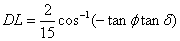(2)

Altitude Angle: (α)

The actual location of sun in the sky at particular place and time is given by equation (3)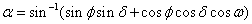(3)

Azimuth angle: (γ)

The surface azimuth of plane should track sun azimuth angle which is given by the equation (4)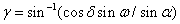(4)

Incident Angle (θ)

The angle of incidence of solar rays on plane of surface considered should be perpendicular to it and should be parallel to perpendicular drawn to the considered surface. This incidence angle is given by (5)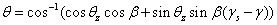(5)

Where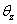is Zenith angle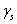is Sun Azimuth angle

γ is Surface Azimuth angle

Zenith angleThe of Sun rays subtend an angle with respect to the Polar axis of earth is known as Zenith angle. It can be from the below equation (6)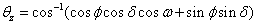(6)

Necessary corrections to available radiation data of considered location

Solar Radiation data which is divided into three major groups i.e., direct or beam radiation, diffuse radiation, Total solar Radiation. Total solar radiation is sum of direct, diffuse, terrestrial radiation. Solar Radiation data plays a vital role in estimating electrical power output from the installed capacity if we want to simulate by using software, the radiation data which can be obtained from IMD on payment basis which is horizontal data need to be corrected if the considered plane is tilt with some angle. Here considered IMD’s relations and Mani’s relations are consider to find total solar radiation which are obtained from the report Solar Radiant Energy over India .

Relationships given by IMD: The Total Solar Radiation GT falling on inclined surface has three components. They are direct solar radiation or Beam GB, diffuse solar radiation GD and reflected solar radiation GR which are measured in W/m2 i.e., Power, if we integrate for specific time then in J/m2 i.e., Energy.

GT= GB+GD↓+GR↑ (7)

Beam Radiation GB of Inclined surface is given by GBH which is beam radiation on horizontal surface.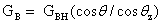Mani’s Relation Ship between global solar radiation on horizontal surface and global solar radiation on horizontal surface. Tilt Factor: KI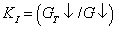(8)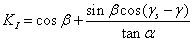(9)

Where G is Global Solar Radiation on Horizontal Surface GT is Total Solar Radiation on tilted Surface GD is diffuse solar radiation on horizontal surface β is inclination angle of surface

#### Extraction of Electrical Parameters of Solar Cell

The solar modules are direct current electrical generators in solar photovoltaic power plant. To estimate electrical output from the considered solar photovoltaic power plant using software’s as the direct installation is costly, as manufacturing cost is more. So, before installing solar photovoltaic power plant in a particular location one must be aware of meteorological data of the location which plays very important role in electrical output from the installed plant and the required meteorological data is Radiation data, Wind speed, Temperature which affects the electrical parameters of solar modules. The electrical parameters of solar modules are photo Current Iph, Dark saturations current Io, Ideality Constant A, Series resistance Rs, Shunt Resistance Rp [2,8]. These electrical parameters which are not present in the data sheet given by manufacturer so we need find these parameters in order to estimate electrical output from the consider module at particular location i.e., Radiation, Temperature and Wind speed.

Solar photovoltaic module: Data sheet terminology

The solar photovoltaic module is made of Ns cells connected in series and Np series strings connected in parallel. Thus formed solar photovoltaic module is Electrical DC generator using solar radiation as input is current source. The current is obtained by the photons of light which when fall on the module creates electron-hole pairs this effect is known as photovoltaic effect, which are allowed to flow through the load connected across the module. The flow of electrons through load is possible because electric field created across the junction of each cell because each photovoltaic cell is a diode which forms by combining P-type and N-type material, each diode has breakdown voltage of around 0.6-0.8 (V). Thus voltage of each cell when combines in series in module adds up to form module voltage. From the data sheet of considered photovoltaic module we get Maximum power Pmp, open circuit voltage Voc, short circuit Isc, maximum power point voltage Vmp, maximum power point current Imp, efficiency of module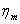, at STC conditions i.e., Gn,T =at 1000 W/m2, Ta=Tc=298 K, air mass 1.5, wind speed Wi=1 m/s, and as well as at NOCT condition i.e., at GT, NOCT =800 W/m2, TNOCT =293 K, air mass 1.5, wind speed Wi=1 m/s. Temperature coefficients of Short circuit current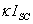, Open circuit Voltage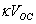, Maximum Power Point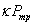, No. of Cells in series and parallel, area of cell and module, weight of the module. By using these values we need to find the above said electrical parameters of considered module to accurately estimate electrical output from the module at particular location and climatic conditions.

Mathematical equations

Electrical parameters of considered solar module as mention above are combine to form basic equation as show below which are obtained from equivalent electrical circuit of solar cell and applying KCL to the circuit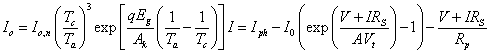(10)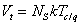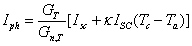(11)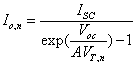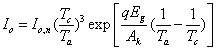(12)

Thermal Voltage at particular cell temperature After seeing these equations we came to know out off five unknown variables we can find two variables Iph,I0 and other three variables need to be calculated those are Rs, Rp, A. Out of these three A which is ideality constant of diode is taken the value between1≤ A ≤ 2 . To get Rs and Rp we need substitute points (Voc,0) open circuit condition, (0,Isc) short circuit condition, (Vmp, Imp) maximum power point condition which we can obtain from data sheet and solve the equation numerically to get unknown variable and this process is trial and error because here A is assumed, final values of unknown variables can be obtained by observing V vsI and V vsP characteristics of the module using Matlab program. The minimum and maximum values of Rs and Rp are obtained respectively using below equations .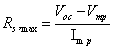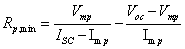Finding cell temperature of module which is different from ambient temperature which plays important role in simulating electrical output from the photovoltaic module which was designed in Matlab/Simulink. The relation between cell and ambient temperature is given by the below equation.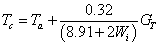Or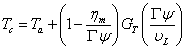where Γ is transmittance of cover over cell ψ is fraction of radiation incident on the surface to the observed υL is loss coefficient i.e., by convection and radiation from the surface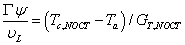Data sheet values of different solar modules

From the below data sheet values given in Tables 1 and 2 and numerical analysis using Matlab program given by Figure 6 and comparing with I-V, P-V characteristics of data sheet and plot obtained from program written in Matlab accurate values of A, Rs and Rp are tabulated below Table 3 - A2 as Iph and Io varies with radiation, Temperature of the cell which depends on ambient temperature and wind speed .

Parameters Tata Solar Titan Solar Vikram Solar Surana solar
Pmp W 250 250 250 250
Voc V 37. 37.80 37.45 37.8
Iac A 8.71 8.63 8.70 8. 63
Vmp V 30.2 30.72 30.58 30.72
Imp V 8.3 8.14 8.18 8.14
?m 0.15 - 0.153 -
Ns 60 60 60 60
?Pmp -1.095 -1.075 -1.025 -1.025
?V oc -0.123 -0.120 -0.116 -0.120
?Isc 0.0055 0.00345 0.00504 0.00345
Area m2 1.460 1.460 - 1.460
TcNOCT K 320 318 318 -
Bipass Diodes - 3 3 3

Table 1: Data sheet values of different manufactures at stc.

Parameters Photon Solar Jupiter solar EMMVEE solar
Pmp W 250 250 250
Voc V 43.06 37.0 37.62
Iac A 7.74 8.87 8.76
Vmp V 36.07 30.5 29.76
Imp V 6.93 8.3 8.40
?m 0.129 0.16 0.154
Ns 72 60 60
?Pmp -1.075 -1.05 -1
?V oc -0.142 -0.1073 -0.127
?Isc 0.00448 0.00354 0.00438
Area m2 1.752 - 1.46
TcNOCT K - 318.5 319
Bipass Diodes - - 3

Table 2: Data sheet values of different manufactures at stc.

Manufacturer Idelity constant A Rs? Rp?
TATA Solar 1.3 0.1165 1444.9
Vikram Solar 1.154 0.2 810
Titan Solar 1.14 0.23 1290
Surana Solar 1.138 0.23 1176
Photon Solar 1.64 0 919.905
Jupiter Solar 1.52 0.3 875
EMMVEE Solar 1.2 0.23 1449

Table 3: Calculated electrical parameters of different modules.

Figure 8: Comparison of power output for month April using 1986-2000 data and 2015 April data.

Figure 9: Estimating electrical energy output from 110.25 kw solar PV system using 1986-2000 data.

#### Meteorological data and electrical energy output estimation

Meteorological data which plays important role in estimating electrical output from solar photovoltaic power plant which was not installed but simulated using Matlab/Simulink software. In this paper author considered data of Radiation on tilt surface with tilt angel of β =17.7 because it gives maximum output for fixed mounting of solar photovoltaic module. This data consists of monthly average daily hourly average instantaneous radiations, temperature, wind speed from year 1986-2000  and comparing with meteorological data collected for month April 2015  at considered location and comparing simulation results electrical energy output for month April and total year using above data from installed 110.25 KW solar photovoltaic power plant. The yearly electrical energy output at particular location is compared with other software’s like PVsyst, SAM, PVwatts and with program developed in this paper values given in Tables 4-6 .

#### Cost Estimation

Solar Photovoltaic Power Plant installation is more costly compare to conventional power plants like Thermal, nuclear, Gas and Oil. But operating cost of solar photovoltaic power is negligible compare to conventional power plants. More over there is no pollution and hence known as green power. By proper sizing and selecting location with care can make comparison cost of energy generation from solar plant with conventional power plants. Electrical Energy generated from the solar power plant is not continuous and thus limits to replace conventional power plants. From Table 7 it was known that considered load consumes 16,57,614 units of electrical energy per year, hourly demand of load is not known as the load is educational institute it works for 10 hours a day and with working days around 240 working days, per day energy consumption on an average is around 6906.72 units, per hour around 690.67 units. To generate 690.67 units per hours solar photovoltaic power plant capacity should be around 1.5 MW, by considering energy output from 1 KW is around 4.8-5.13 units per day at considered location i.e., Andhra university, Visakhapatnam. Initial cost is more and more over educational institutes will get 30% subsidy on 100 KW solar photovoltaic power plant from government of India [13,14]. To estimate cost of electrical energy per unit we need to know solar photovoltaic power plant equipment used and there cost. According to Government of India bench mark cost for 1 MW solar photovoltaic power plant for the year 2015-2016 is Rs 605.85 Lakhs. Which include Photovoltaic Modules, Land cost, civil and general works, mounting structures, Power conditioning unit, Cables and Transformers, Preliminary and Pre-operative Expenses. Photovoltaic panel costs around 55% of total cost so one must be careful in choosing photovoltaic panes. From the above benchmark cost for 1 MW we can estimate cost for 110: 25 KW i.e., around Rs 58.733 lakhs. Now in this paper, considered load College of Engineering, Andhra University which consumes electrical energy as shown in Table 8 [15-17].

Source Electrical Energy Output
Matlab data(1986-2000) 20395 KWh
Matlab data(April 2015) 17605 KWh
PVwatts 17444 KWh
PVsyst 17130 KWh
SAM 18320 KWh

Table 4: Electrical energy produced from 110.25 kw for the month April of considered location.

Source Electrical Energy Output
Matlab 159818 KWh
PVwatts 175266.32 KWh
PVsyst 179770 KWh
SAM 195724 KWh

Table 5: Electrical energy produced from 110.25 kw for year of considered location.

Year Rs/KVAh Rs/KVA Bill paid (Rs)
2013-14 6.90 350 -
2014-15 6.90 350 1,52,58,163
2015-16 7.31 371 -

Table 6: Tariff per kvah paid to conventional utility.

Year MD(KVA) Units(KVAh) Rs/KVAh
2014-15 650.46(700) 1657614 9.204

Table 7: Yearly electrical energy consumed by considered load.

KVAh generated Total Cost(Rs) Rs/KVAh
3579930 11754295 3.8

Table 8: Life time energy output from installed 110.25 kw Solar Photovoltaic power plant.

#### Factors Determining Solar Plant Feasibility

Performance Ratio, Capacity Utilization Factor, LCOE are important factors to compare photovoltaic power plant with conventional power plants . Performance Ratio is the ratio of energy generated or measured to energy modelled. This parameter is used to compare the actual plant performance which used to rectify fault which decrease energy output from the installed system.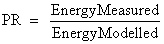(14)

Capacity Utilization Factor is the ratio energy output from installed system to the energy it would have generated if the system is available for 24 hours and 365 days.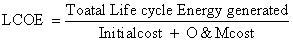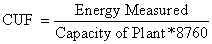(15)

Levelized Cost of Electricity is used to compare cost per unit of non-conventional system with conventional system. It is the ratio of total life cycle cost to total lifetime energy production. Total life cost includes initial cost, operation and maintenance cost.(16)

From Table 7 it is known how much money paid by the consumer to the utility and units consumed by considered Load. If for the considered load 110.25 KW solar photovoltaic power is installed, in this paper comparison was made between retailer price and renewable energy source unit price . To calculate cost of generation of one unit electrical energy from the installed load and payback period, Performance ratio and capacity utilization factor of installed solar photovoltaic plant. Solar photovoltaic power life time is considered 25 years in which it yields 90% of electrical energy for first 10 years and then up to 25 years it yields about 80% comparing with first years output. Operation and maintenance cost will be around Rs 1.3 lakhs [20,21].

#### Conclusion

Estimation of electrical power output from Solar Photovoltaic Power Plant at particular location using Matlab programme requires accurate calculation of five parameters of solar cell, calculation of radiation data on tilted surface and knowing location temperature and wind speed (Figures 7-10). In this paper 110.25 KW solar photovoltaic power plant is considered and using radiation data of Visakhapatnam from year (1986-2000) and radiation data of the month April 2015 to calculate AC energy output and compare these results with PVwatts, PVsyst, SAM. The calculated values of LCOE is 3.8 Rs/KVAh given in Table 5 if plant operates for 25 years and first year energy Production was 159818 KVAh and for 25 years the energy output is 3579930 KVAh.

#### References

Select your language of interest to view the total content in your interested language

### Article Usage

• Total views: 15360
• [From(publication date):
March-2016 - Jan 25, 2020]
• Breakdown by view type
• HTML page views : 14834Can't read the image? click here to refresh